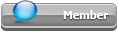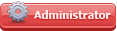## Featured Articles

Check out the latest featured articles.## File Library

Check out the latest downloads available in the File Library.2# Line Sizing: Pressure Drop/ Minor Losses Coefficient Of Valves, Fittin

pressure drop line sizing resistance coefficient minor losses valves & fittings resistance minor losses table minor losses calculations

4 replies to this topic
Share this topic:
|

### #1zavtranguyen

zavtranguyen

Junior Member

•• Members
•• 14 posts

Posted 10 May 2021 - 09:43 PM

Hi every one, in "line sizing" when we caclulate the item "pressure drop", for such minor losses as of valves and fittings, resistance coefficients k should be taken from tables. Howerver, i see that we can also use formula to get those k and i have some formulas for this purpose, but i cann't check the rightness of those formulas, or any one could help me to confirm from which handbooks/documents the formulas were taken.

Please find the formulas below

Valves and fittings (PNS = pipe norminal size, inch)

Formula

1. Pipe entrance

k= 0.5

2. Pipe exit

k= 1

3. Elbow 90

• Re<500000:

k =0.00476*(ln(Re))^2-  0.1574*ln(Re)*1.446+(Re/500000)^0.25*(0.01163*(ln(e/D))^2+0.2278*ln(e/D)+1.1055)

• Re>=500000:

K = 0.01163*(ln(e/D))^2+0.2278*ln(e/D)+1.3055)

4. Tee turn

k=1

5. Globe v

• PNS > 26”: k=4
• PNS<=26”: k= 10^(-0.2258*log(PNS)+0.9)

6. GATE V

• PNS > 26”: k = 0.1
• 5”< PNS <=26”: k = 10^(-0.1542*log(PNS)-0.7782)
• PNS < 5”: k = 10^(-0.2382*log(PNS)-0.7195)

7. CHECK V

• PNS >= 20”: k = 0.6
• 2” <PNS < 20: k = 10^(-0.1856*log(PNS)+0.01967)
• PNS <2”: 10^(-0.2534*log(PNS)+0.3551)

8.. BUTT V

• PNS> 22”: k= 0.3
• 6”<PNS<= 22”: k= 10^(-0.6523*log(PNS)+0.3527)
• PNS<= 6”: k= 10^(-0.2287*log(PNS)+0.02307)

9. BALL V

k=1.5

Thanks a lot.

Edited by zavtranguyen, 10 May 2021 - 10:00 PM.

### #2breizh

breizh

Gold Member

•• Admin
•• 5,022 posts

Posted 10 May 2021 - 10:52 PM

Hi,

Consider the link attached https://neutrium.net...-head-k-method/

together with the paper from Hooper .

Use the link above to learn about 2K and 3K methods. , Get a copy of Crane Flow of fluids through valves, fittings and pipe TP N 410

Good luck

Breizh

### #3zavtranguyen

zavtranguyen

Junior Member

•• Members
•• 14 posts

Posted 11 May 2021 - 08:33 PM

Thank you for your valuable information, Mr.Breizh.

### #4latexman

latexman

Gold Member

•• Admin
•• 1,135 posts

Posted 11 May 2021 - 08:53 PM

For:
“9. BALL V
k=1.5”

I think k = 0.1 for full port and k = 0.2-0.5 for reduced port. k = 1.5 for ball valve is too large.

### #5zavtranguyen

zavtranguyen

Junior Member

•• Members
•• 14 posts

Posted 12 May 2021 - 12:31 AM

Thank you Mr.Latexman, it's really too large for ball valve, so i have some doubts that whether these formulas are corret or not.